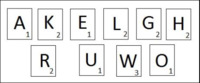# Need help understanding this problem

#### Tangentjay

##### New memberWe have this picture and the questing is:
What is the probability the tile is worth 3
points given the tile is a consonant?

and
A player selects two tiles blindly without replacement. What is the probability the
second tile is a vowel given the first tile is a consonant?

Is this like the Urn example with ball replacement? would I solve it the same way? Any examples would be nice. Thank you.

Last edited:

#### kwatts59

##### New member
There are six consonants, K, L, G, H, R and W, only 1 of them (the W) is worth 3 points.
Given that a consonant tile was selected, what is the probability that it is a W?
The answer is 1/6 or .16667 or 16.667%.

There are 10 tiles, the first tile selected is a consonant. There are 9 tiles left, 4 of which are vowels, A, E, U and O.
The probability that the second tile selected is a vowel is 4/9 or 0.44444 or 44.444%.

#### Tangentjay

##### New member
There are six consonants, K, L, G, H, R and W, only 1 of them (the W) is worth 3 points.
Given that a consonant tile was selected, what is the probability that it is a W?
The answer is 1/6 or .16667 or 16.667%.

There are 10 tiles, the first tile selected is a consonant. There are 9 tiles left, 4 of which are vowels, A, E, U and O.
The probability that the second tile selected is a vowel is 4/9 or 0.44444 or 44.444%.

Ty for the answer, but I was wondering if it was like the Urn probability? Would I tackle the question the same way?

#### lookagain

##### Elite Member
The answer is . . .

kwatts59, do not provide solutions to posters who have just posted problems, especially with
no work shown. Provide hints and/or prompts for them.

#### Jomo

##### Elite Member
View attachment 26462

We have this picture and the questing is:
What is the probability the tile is worth 3
points given the tile is a consonant?

and
A player selects two tiles blindly without replacement. What is the probability the
second tile is a vowel given the first tile is a consonant?

Is this like the Urn example with ball replacement? would I solve it the same way? Any examples would be nice. Thank you.
Sure it is like an urn problem. Just imagine that the tiles are in a urn.

#### Tangentjay

##### New member
kwatts59, do not provide solutions to posters who have just posted problems, especially with
no work shown. Provide hints and/or prompts for them.
Yes, I was wonder if I would try this out by what JoMo said, Imagine that the tiles are in a urn haha.

#### Tangentjay

##### New member
Also I think this would be a Conditional Probability.

My question was:
player selects a single letter tile blindly. What is the probability the tile is worth 3
points given the tile is a consonant?

I think it would be P(1/10|1/6)

because there are ten total and I'm going in blind, Kwatt said "
There are six consonants, K, L, G, H, R and W, only 1 of them (the W) is worth 3 points.
Given that a consonant tile was selected, what is the probability that it is a W?
The answer is 1/6 or .16667 or 16.667%. "

Am I on the right track? thank you.

#### Tangentjay

##### New member
Also I think this would be a Conditional Probability.

My question was:
player selects a single letter tile blindly. What is the probability the tile is worth 3
points given the tile is a consonant?

I think it would be P(1/10|1/6)

because there are ten total and I'm going in blind, Kwatt said "
There are six consonants, K, L, G, H, R and W, only 1 of them (the W) is worth 3 points.
Given that a consonant tile was selected, what is the probability that it is a W?
The answer is 1/6 or .16667 or 16.667%. "

Am I on the right track? thank you.

The answer is 100% if anyone wanted to know. I figured it out

#### Tangentjay

##### New member
The answer is 100% if anyone wanted to know. I figured it out
Sorry, I don't know how to edit, but the answer is 100% because P(3 points/cons)

I'm afraid not.